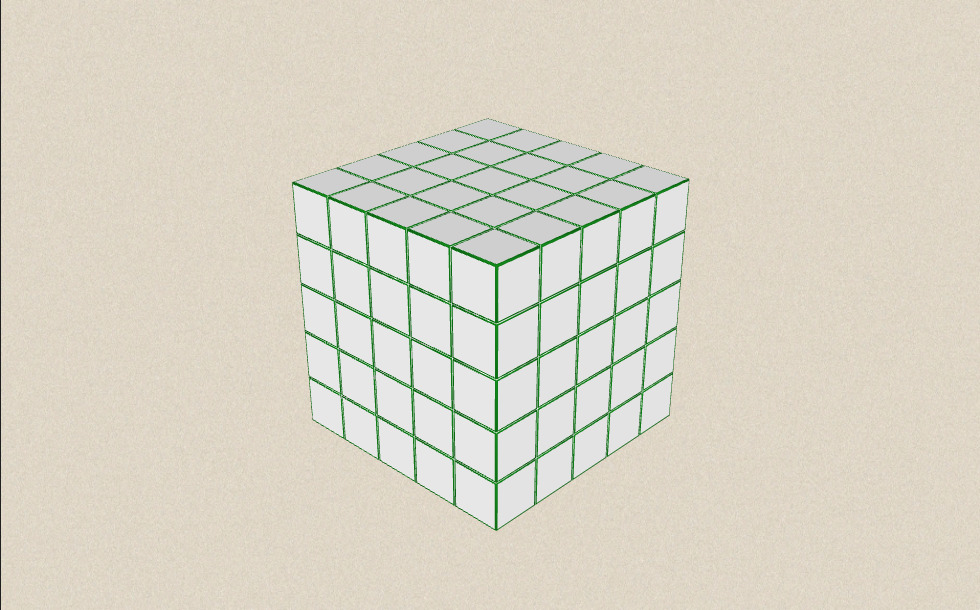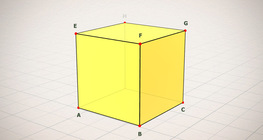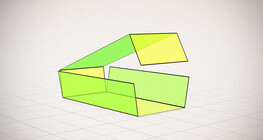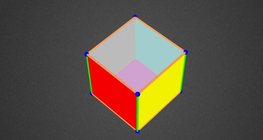Дана: 0

Барлыгы: 0,00

0

# Volume and surface area (exercise)

### Volume and surface area (exercise)

An exercise about the volume and surface area of solids generated from a ´base cube´.

Математика

Этикеткалар

куб, көлем, surface, exercise

Байланысты экстралар

### Көріністер### Байланысты экстралар#### Cube

This animation demonstrates the components (vertices, edges, diagonals and faces) of the...#### Cube puzzle

Building cubes shown in several views from the available unit cubes aids spatial vision...#### Жазық фигуралардың периметрі мен ауданын, беттің ауданын және көлемін есептеу

Анимацияның көмегімен жазық фигуралардың периметрі мен ауданын есептеу формуласымен,...#### Қысқаша көбейтудің формулалары

Анимацияда қысқаша көбейту формулаларының ұқсас қосылғыштары көрсетіледі.#### Building shapes (one colour)

Build 3D shapes from unit cubes with the help of several views.#### Cube (exercises)

Edges, diagonals and faces of a cube can be identified by its vertices.#### Cube sections (exercise)

Examining solids formed by the intersection of a cube and a plane.#### Cuboid

A cuboid is a polyhedron with six rectangular faces.#### Net of a cube (exercises)

Not all nets consisting of 6 congruent squares are foldable into cubes.#### Nets of a cuboid (exercises)

This animation demonstrates the different nets of a cuboid and includes a game.#### Ratio of volumes of similar solids

This 3D scene explains the correlation between the ratio of similarity and the ratio of...#### Volume of spheres (Cavalieri´s principle)

Calculating the volume of a sphere is possible using an appropriate cylinder and cone.#### Volume of spheres (demonstration)

The sum of the volume of the ´tetrahedrons´ gives an approximation of the volume of the...#### Colouring a cube

Colouring the vertices, edges and faces of a given cube according to the criteria...#### Cube of cubes

An exercise about the regular hexahedron built from unit cubes to help deepen your...#### Grouping of cuboids

This animation demonstrates various types of cuboids through everyday objects.#### Non-orientable surfaces

The Möbius strip and the Klein bottle are special two-dimensional surfaces with only one...